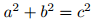# 0-0: Browser Test

This site uses different mechanisms for displaying LaTeX in your browser. If they are all working, the following lines should show three copies of the same familiar equation (with some slight style differences):$a^2+b^2=c^2$

$$a^2+b^2=c^2$$These are course notes for the University of Waterloo's course Math 600: Mathematical Software.
© 2012—. Written and developed by David Pritchard and Stephen Tosh. Contact (goes to the CEMC)